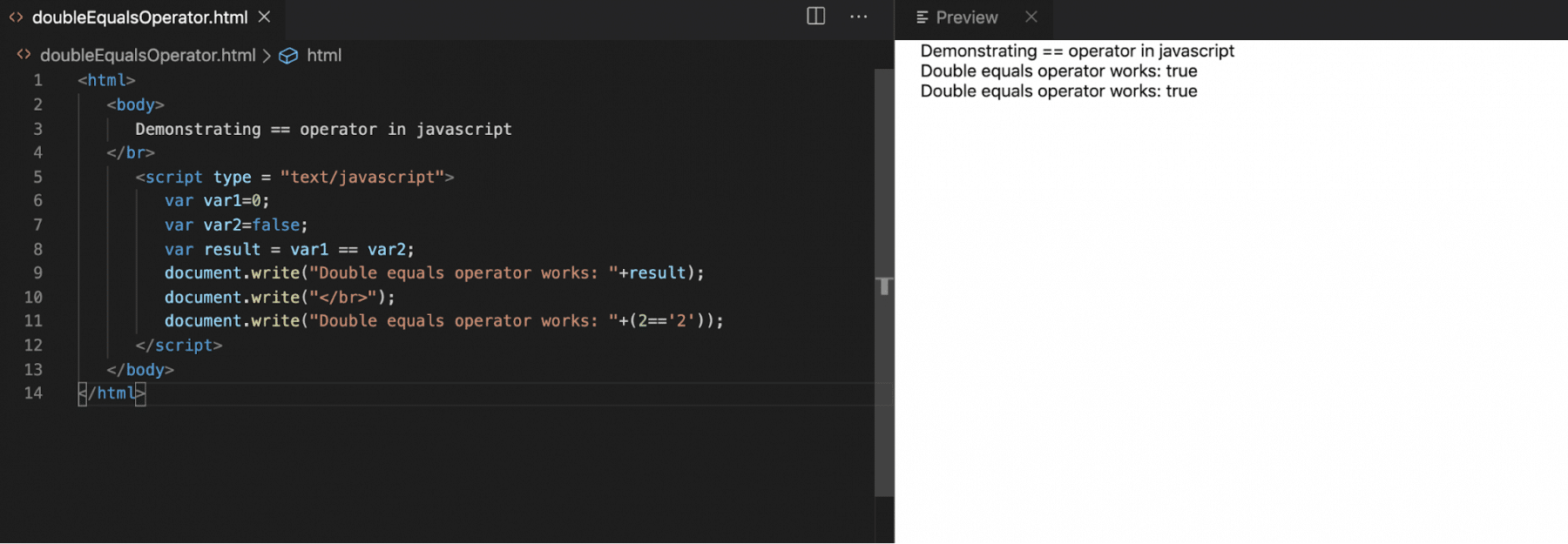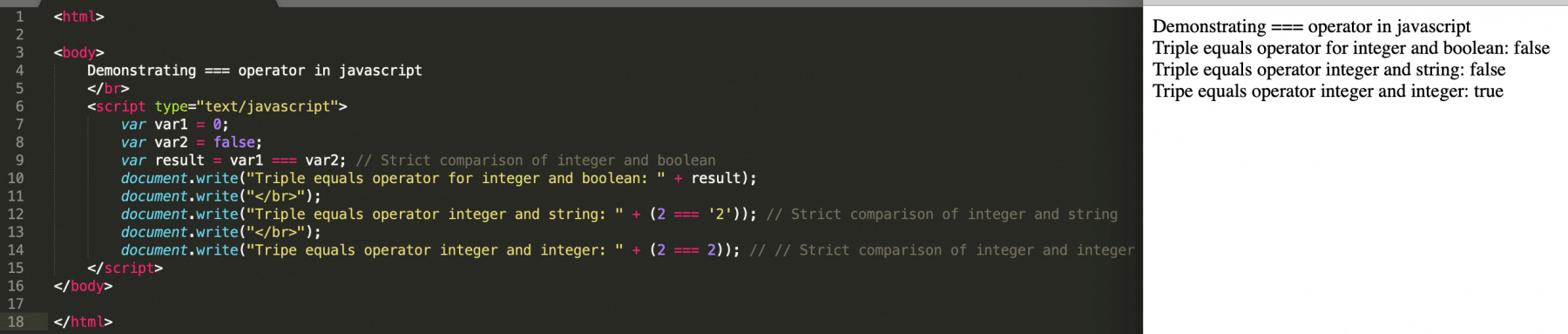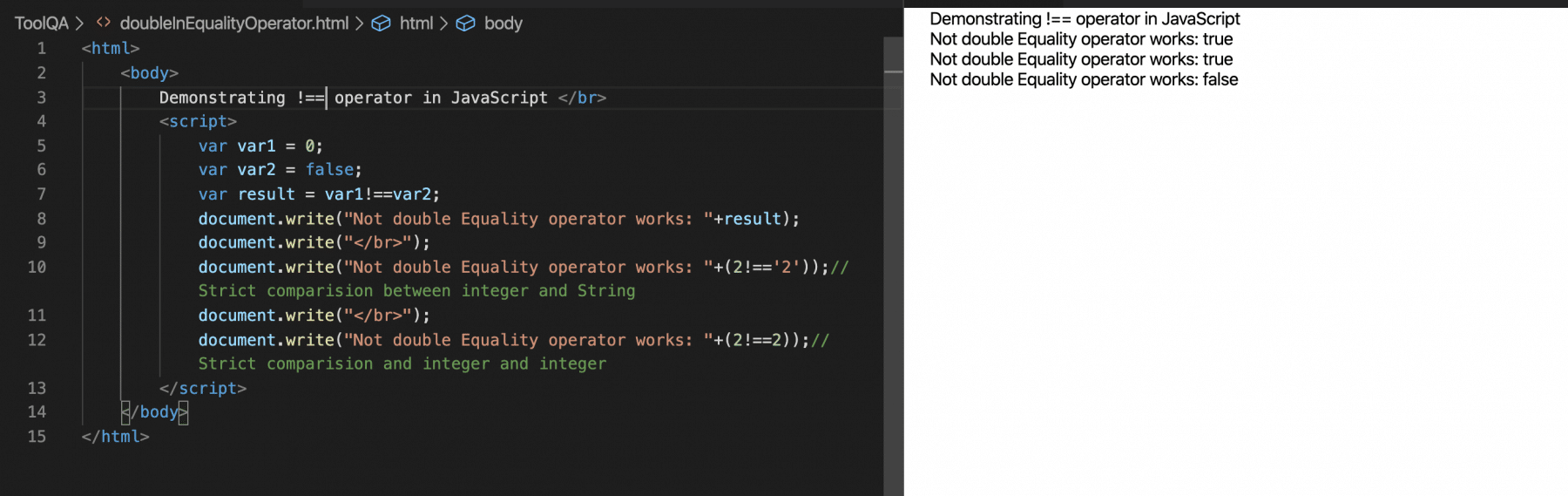Comparing any two values for equality/inequality is one of the basic functioFnalities provided by any programming language. Each programming language provides some operators to compare all kinds of operands. JavaScript also inherits and extends the same concept and provides two variants of operators for comparing whether two operands are equal or not. In this article, we will discuss the Equality operator in JavaScript as well as the Inequality operator along with their differences. We will also cover the below topics:

• What is an Equality operator ( == ) in JavaScript?
• Definition of Identity / strict equality (===) operator?
• What is an InEquality operator ( != ) in Programming?
• What is Identity / strict Inequality (!==) operator?

## What is Equality (==) operator in JavaScript?

In Javascript, the '==' operator is also a loose equality operator that mainly compares two values on both sides and then return true or false.

Its syntax looks like below:

Syntax:

``````operand1 == operand2
``````

It is type coercion, which means that the two values are compared only after attempting to convert them into the same type. If there is a type mismatch between the operands, it first converts the operands to the same type if they are not of the same type, then applies strict comparison. If both operands are objects, then, in this case,  JavaScript carries out a comparison of internal references, which are equal when operands refer to the same object in memory. Let's understand these details with the help of the following examples:

Example1:

``````var var1 = 5;
var var2 = 5;
document.write(var1 == var2);//Output will be true
``````

In the above example, we have taken variables that are of both number type. When we compare them using the "==" operator, JavaScript will just compare the values directly and will not do any type of conversion.

Example2:

``````var var1 = 5;
var var2 = '5';
document.write(var1 == var2);//output will be true

``````

In the above example, var1 is of number type, and var2 is of string type. Before comparing both the variables, JavaScript will first convert the types of variables, so both the variables have the same type. In this example, it will convert the string variable to a number and then will make the comparison of both the numbers. So, ideally, it just compares the values of the variables and not the data type of the variable.

The complete working functionality of the equality operator is as below with the help of the following example:

``````<html>
<body>
Demonstrating == operator in javascript
</br>
<script type = "text/javascript">
var var1 = 0;
var var2 = false;
var result = var1 == var2; // Comparing an integer and boolean
document.write("Double equals operator works: " + result);
document.write("</br>");
document.write("Double equals operator works: " +(2 == '2')); // Comparing an integer and string
</script>
</body>
</html>
``````

Save the file with name  doubleEqualOperator.html and open it in any browser (Chrome, Firefox, or IE). It should show the output as:In the above example, we can see that var1 = 0 and var2 = false when comparing this, the compiler first converts var2(false) value to 0 and then compares the values.

Similarly, for the other case on line number 12, the compiler first converts the string value '2' to number 2 and then performs the comparison.

## What is Identity / strict equality (===) operator?

The triple equals (===) is called a strict equality or identity operator. Its syntax looks like below:

Syntax:

``````operand1 === operand2
``````

The result of the identity operator is true if the operands are strictly equal with no type-conversion. During this comparison, the compiler doesn't convert the value implicitly; instead of this, it will compare both the value and data type of the operands. Let's understand these with the help of the following examples:

Example1:

``````var var1 = 5;
var var2 = 5;
document.write(var1 === var2);//output will be true
``````

In the above example, we have taken variables both variable "var1" and "var2" of number types. In this case, as both the values of variables and their datatype are the same, JavaScript will return the result of comparison true.

Example2:

``````var var1 = 5;
var var2 = '5';
document.write(var1 === var2);//output will be false
``````

In the above example, we have taken var1 is of number type, and var2 is of string type. In this case, === operator is not only checking the value but also the datatype, and as the data type of both the variables is not the same, the output will be false.

The detailed working functionality of the strict equality operator is as shown below, with the help of the following example.

``````<html>

<body>
Demonstrating === operator in javascript
</br>
<script type="text/javascript">
var var1 = 0;
var var2 = false;
var result = var1 === var2; // Strict comparison of integer and boolean
document.write("Triple equals operator for integer and boolean: " + result);
document.write("</br>");
document.write("Triple equals operator integer and string: " + (2 === '2')); // Strict comparison of integer and string
document.write("</br>");
document.write("Tripe equals operator integer and integer: " + (2 === 2)); // // Strict comparison of integer and integer
</script>
</body>

</html>
``````

Save the file with name tripleEqualOperator.html and open it in any browser (Chrome, Firefox, or IE). It should show the output as:In the above example, we can see that var1 = 0 and var2 = false when comparing this, the compiler compares both values, as well as the data type of the variables and no implicit conversion of datatype, happens during the comparison. As the screenshot clearly shows, the operator returns true only when both the value as well as the data type of the operands are the same.

## What is InEquality (!=) operator?

In Javascript, the '!=' operator is known as a loose inequality operator, which mainly compares two values on both sides and then returns true if the values are not equal and false if the values are equal.

Its syntax looks like below:

Syntax:

``````operand1 != operand2
``````

Similar to the Equality operator, this operator also converts the type of both the operands if needed. If there is a type mismatch between the operands, It first converts the operands to the same type and then applies comparison. Let's understand this with the help of the following examples:

Example1:

``````var var1 = 5;
var var2 = 4;
document.write(var1 != var2);//Output will be true
``````

In the above case, we have taken variables, both of which are of number type. When we compare them using the "!=" operator, JavaScript will just compare the operands based on their values. In this scenario, no type-conversion happens, as both the operands are of the same type.

Example2:

``````var var1 = 5;
var var2 = '5';
document.write(var1 != var2);//output will be false

``````

In the above example, var1 is of number type, and var2 is of string type. Before comparing both the variables, JavaScript will first convert the data type of variables, so that both the variables have the same dataType. In this example, it will convert the string variable to the number and then will make the comparison of both the numbers. So, ideally, it just compares the values of the variables and not the data type of the variable. As the value of both the variables is 5, so the "!=" operator will return result as false.

The detailed working functionality of the inequality operator is as shown below, with the help of the following example:

``````<html>

<body>

Demonstrating != operator in javascript

</br>

<script type = "text/javascript">

var var1 = 1;

var var2 = false;

var result = var1 != var2; // Comparing an integer and boolean

document.write("In Equality operator works: " + result);

document.write("</br>");

document.write("In Equality operator works: " + (2 != '2')); // Comparing an integer and string

</script>

</body>

</html>

``````

Save the file with name  doubleInEqualOperator.html and open it in a browser. It should show the output as:In the above example, we can see that var1 = 1 and var2 = false when comparing this, the compiler first converts var2(false) value to zero(0) and then compares the values. Similarly, for the other case at line 10, the compiler first converts the string value '2' to number 2 and then performs the comparison.

## What is Identity / strict inequality (!==) operator?

The not double equals (!==) is called a strict inequality operator. If the operands are strictly unequal with no type-conversion then the identity operator results true. During this comparison, the compiler doesn't convert the dataType of operands implicitly. Instead of this, it will compare both the values as well as the data type of operands. Its syntax looks like below:

Syntax:

``````operand1 !== operand2
``````

Let's understand this with the help of the following examples:

Example1:

``````var var1 = 5;
var var2 = 5;
document.write(var1 !== var2);//output will be false
``````

In the above example, we have taken variables both variable "var1" and "var2" of number types. In this case, as both the values of variables and their datatype are the same, JavaScript will return, the result of the comparison is false.

Example2:

``````var var1 = 5;
var var2 = '5';
document.write(var1 !== var2);//output will be true
``````

In the above example, we have taken var1 is of number type, and var2 is of string type. In this case !== operator is not only checking the value but also the datatype, and as the data type of both the variables is not the same, the output will be true.

The working functionality of the strict inequality operator is as shown below, with the help of the following example.

``````<html>
<body>
Demonstrating !== operator in JavaScript </br>
<script>
var var1 = 0;
var var2 = false;
var result = var1!==var2;
document.write("Not double Equality operator works: " + result);
document.write("</br>");
document.write("Not double Equality operator works: " + (2! == '2'));//Strict comparision between integer and String
document.write("</br>");
document.write("Not double Equality operator works: " + (2! == 2));//Strict comparision and integer and integer
</script>
</body>
</html>
``````

Save the file with name notDoubleEqualOperator.html, and open it in any browser (Chrome, Firefox, or IE). It should show the output as:In the above example, we can see that var1 = 0 and var2 = false when comparing this, the compiler compares both values, as well as the data type of the variables and no implicit conversion of datatype, happens during the comparison. As the screenshot clearly shows, the operator returns false only when both the value as well as the data type of the operands are the same.

## Key Takeaways

• The equality(==) and Inequality(!=) operators compares the operands only based on their values and ignores the datatypes of the operands.
• The strict equality(===) and strict inequality(!==) operators compare the operands based on both values as well as datatypes of the operands.

I hope, you got a fair amount of understanding about Javascript Equality Operator, Inequality operator as well as Strict Equality and Strict Inequality Operator from this article. Further, let's move to the next article, in which we are going to learn about "Arrays" in JavaScript.